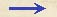Discorsi Propositions 1/03-th-03 Discorsi Proposition1/03-th-03THEOREMA III, PROPOSITIO III. THEOREM III, PROPOSITION IIIInaequalibus velocitatibus per idem spatium latorum tempora, velocitatibus e contrario respondent. In the case of unequal speeds, the time-intervals required to traverse a given space are to each other inversely as the speeds. Sint velocitates inaequales A maior, B minor, et secundum utramque fiat motus per idem spatium CD: dico, tempus quo A velocitas permeat {194} spatium CD, ad tempus quo velocitas B idem spatium permeat, esse ut velocitas B ad velocitatem A.Fiat enim ut A ad B, ita CD ad CE; erit igitur, ex praecedenti, tempus, quo A velocitas conficit CD, idem cum tempore quo B conficit CE: sed tempus quo velocitas B conficit CE, ad tempus quo eadem conficit CD, est ut CE ad CD; ergo tempus quo velocitas A conficit CD, ad tempus quo velocitas B idem CD conficit, est ut CE ad CD, hoc est ut velocitas B ad velocitatem A: quod erat intentum. {10} Let the larger of the two unequal speeds be indicated by A; the smaller, by B; and let the motion corresponding to both traverse the given space CD. Then I say the time required to traverse the distance CD at speed A is to the time required to traverse the same distance at speed B, as the speed B is to the speed A. For let CD be to CE as A is to B; then, from the preceding, it follows that the time required to complete the distance CD at speed A is the same as [I94] the time necessary to complete CE at speed B; but the time needed to traverse the distance CE at speed B is to the time required to traverse the distance CD at the same speed as CE is to CD; therefore the time in which CD is covered at speed A is to the time in which CD is covered at speed B as CE is to CD, that is, as speed B is to speed A. Q. E. D.Discorsi Propositions 1/03-th-03 Discorsi Proposition1/03-th-03